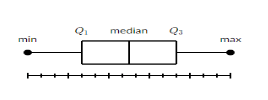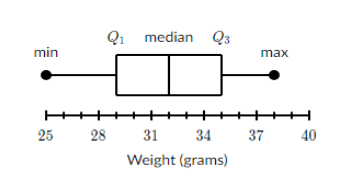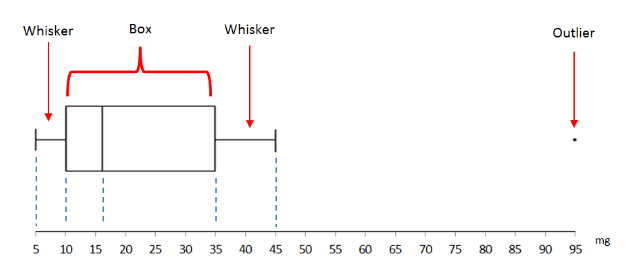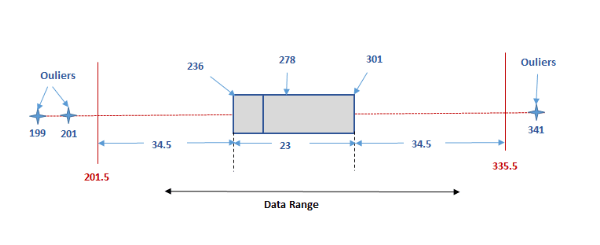# Box and Whiskers Diagrams

## Summary

• A box and whiskers diagram displays a summary of a set of data.
• Maximum, Minimum, First Quartile, Third Quartile and Median, interquartile, upper limit, lower limit.
• Outlier can be present in the measurement, they thus need to be shown separately on the box and whiskers diagram.

A box and whiskers diagram is also known as box plot, it displays a summary of a set of data. Minimum, maximum, median, first quartile and third quartile, interquartile, upper limit and lower limit. It has many advantages:

• Two or more data can be compared
• It is less costly
• Saves a lot of time
• Easy to draw and understand
• We can study the shape of the data and discover the relation between mean, median and mode
• Provides indication of data’s symmetry and skewness
• It shows outlier#### Example #1

A sample of 1010 boxes of raisins has these weights (in grams):

28, 25, 29, 29, 35, 34, 30, 35, 37, 38

In order to draw a box plot we first need to find out the maximum, minimum, quartiles and the median. We will first put the data in the order from the smallest number to the largest number.

25, 28, 29, 29, 30, 34, 35, 35, 37, 38

Then to find the maximum value we will find the largest number in the dataset which in this case is 38.

Similarly for the smallest number we will look for the smallest number in the dataset, which in this case is 25.

We will then find the median in the data, which will require the formula that we studied in the previous chapter.

Since n = 10 in this set thus, the median will not be explicit and so we will take the midpoint of the two middle values:$\frac { 30\quad +\quad 34 }{ 2 } \quad =\quad 32$

The median is 32.

To find our Median we can also use the formula:${ Q }_{ 2 }\quad =\quad \frac { 1 }{ 2 } { (n\quad +\quad 1) }^{ th }\quad term$

Next we will find out both the first and the third quartiles .

The first quartile is the middle value to the left of the median in the data set.

25, 28, 29, 29, 30

So the middle value of this data is${ Q }_{ 1 }\quad =\quad 29$.

We can also figure out the value of the quartiles through a formula for the lower quartile (${ Q }_{ 1 }$):${ Q }_{ 1 }\quad =\quad \frac { 1 }{ 4 } { (n\quad +\quad 1) }^{ th }\quad term$

Similarly, the third quartile will be the middle value to the right of the median in the data set:

34, 35, 35, 37, 38

The middle value of this data is${ Q }_{ 3 }\quad =\quad 35$.

And we can also use a formula to figure out the upper quartile (${ Q }_{ 3 }$):${ Q }_{ 3 }\quad =\quad \frac { 3 }{ 4 } { (n\quad +\quad 1) }^{ th }\quad term$

We can now plot the box and whiskers diagram using all the information above:

Maximum = 38
Minimum = 25
Median =32${ Q }_{ 1 }$  = 29${ Q }_{ 3 }$  =35

To make the box plot we will first draw the number line which fits all the five values above.Next, we will draw the box on top showing both are quartiles, median and the max and min points.#### OutliersHowever, when collecting data it can be possible that some values may be much higher than the other values in the dataset or might be much lower than the dataset. This could be due to variability in the measurement of the data. Such values are known as an outlier they can be excluded from the box and whiskers diagram instead plotted separately, labelled as an outlier. The diagram below shows how it should be shown on a graph.

The first example was an easy example now let’s move onto something slightly more complicated.

The box and whiskers diagram in the exam should be presented in the following way .#### Example #2

Let the data range be:

[199, 201, 236, 269, 271, 278, 283, 291, 301, 303 and 341]

We know that our n = 11 and that our data is already in an ordered form
We further need to find our quartiles, median, minimum and maximum.

For the median:${ Q }_{ 2 }\quad =\quad \frac { 1 }{ 2 } { (11\quad +\quad 1) }^{ th }\quad term\quad =\quad { 6 }^{ th }\quad term$

The${ 6 }^{ th }$  term from our given data set is 278.

The lower quartile:${ Q }_{ 1 }\quad =\quad \frac { 1 }{ 4 } { (11\quad +\quad 1) }^{ th }\quad term\quad =\quad { 3 }^{ rd }\quad term$

The${ 3 }^{ rd }$  term from our data set is 236.

The upper quartile:${ Q }_{ 3 }\quad =\quad \frac { 3 }{ 4 } { (11\quad +\quad 1) }^{ th }\quad term\quad =\quad { 9 }^{ th }\quad term$

The${ 9 }^{ th }$  term from our dataset is 301.

Will now move onto finding our interquartile and then the upper and lower limit.

For the interquartile we will use the following formula:

Interquartile Range (IQR) = Upper Quartile (${ Q }_{ 3 }$) – Lower Quartile (${ Q }_{ 1 }$)

Our upper quartile is 301 and the lower quartile is 236, putting them in the formula we get:

Interquartile Range =  301 – 23 = 23

From the interquartile we can find our upper and lower limit through the following formulas:

Lower Limit =${ Q }_{ 1 }$ – 1.5 IQR.

Upper Limit =${ Q }_{ 3 }$ + 1.5 IQR

So by putting the values in our formula we get:

Lower limit = 236 – 1.5(23) = 201.5

Upper limit = 301 + 1.5(23) = 335.5

We now know that our upper limit is 335.5 and our lower limit is 201.5 and values more than 335.5 and less that 201.5 will be considered as an outlier.

Looking at our data given we already know that 341 is more than 335.5 and both 201 and 199 are less than 201.5 thus all three of these values will be considered as an outlier and will be shown on the graph in the following way.